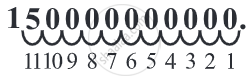# Expressing large numbers in the standard form:

large numbers can be conveniently expressed using exponents.

1. Sun is located 300,000,000,000,000,000,000 m from the center of our Milky Way Galaxy.
2. Number of stars in our Galaxy is 100,000,000,000.
3. Mass of the Earth is 5,976,000,000,000,000,000,000,000 kg.

These numbers are not convenient to write and read. To make it convenient.

Any number can be expressed as a decimal number between 1.0 and 10.0 including 1.0 multiplied by a power of 10. Such a form of a number is called its standard form.

• Step 1: First of all count the number of digits from left leaving only the first digit.
• Step 2: To write it in exponent or standard form, write down the first digit.
• Step 3: If there are more digits in the number then put a decimal after the first digit and then write down the other digits until the zero comes.
• Step 4: Now place a multiplication sign and then write down the counted digits in the first step as the exponent to the base number 10.150,000,000,000 = 1.5 × 1011

While converting a very large number like 150,000,000,000 in a standard form we need to move the decimal place towards the left And when we do so the exponent will be positive.

Thus,

59 = 5.9 × 10 = 5.9 × 101

590 = 5.9 × 100 = 5.9 × 102

5900 = 5.9 × 1000 = 5.9 × 103

5900 = 5.9 × 10000 = 5.9 × 104 and so on.

Thus,

5,985 = 5.985 × 1,000 = 5.985 × 103 is the standard form of 5,985.

Note, 5,985 can also be expressed as 59.85 × 100 or 59.85 × 102. But these are not the standard forms, of 5,985. Similarly, 5,985 = 0.5985 × 10,000 = 0.5985 × 104 is also not the standard form of 5,985.

We are now ready to express the large numbers

The, distance of Sun from the centre of our Galaxy i.e., 300,000,000,000,000,000,000 m can be written as 3.0 × 100,000,000,000,000,000,000 = 3.0 × 1020m

Count the number of zeros in it. It is 10. So, 40,000,000,000 = 4.0 × 1010

Mass of the Earth = 5,976,000,000,000,000,000,000,000 kg = 5.976 × 1024 kg.

#### Example

Express the following number in the standard form: 5985.3

5985.3 = 5.9853 × 1000 = 5.9853 × 103.

#### Example

Express the following number in the standard form: 65,950

65,950 = 6.595 × 10,000 = 6.595 × 104.

#### Example

Express the following number in the standard form: 3,430,000

3,430,000 = 3.43 × 1,000,000 = 3.43 × 106.

#### Example

Express the following number in the standard form: 70,040,000,000

70,040,000,000 = 7.004 × 10,000,000,000 = 7.004 × 1010.

If you would like to contribute notes or other learning material, please submit them using the button below.

### Shaalaa.com

How to Express Natural Number in Exponential Form Using Powers of 10 [00:05:52]
S
0%